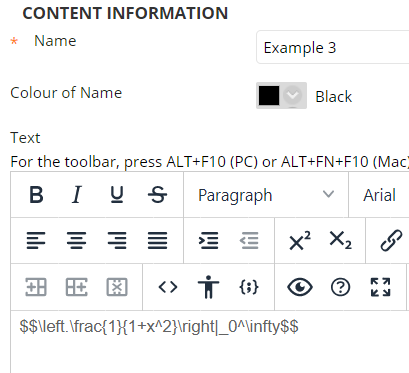# Blackboard – Mathematical notation

Contents

## LaTex in Blackboard

There are a number of ways to use mathematical notation in Blackboard.  This page summarises a technique to use the Display mathematic mode of TeX within Blackboard.

This guide refers to typing and displaying mathematical notation.  This method is not suitable for building formulas for use in Calculated Formula question types.

### Basic operation

When using the Blackboard text editor, for example when creating a content item, or a test question, you can use the $$..$$ TeX primitive syntax.

#### Superscript example

$$e^{e^{e^{e^{x}}}}$$

in Blackboard:

Results in:

In the Blackboard App it looks like this:

##### Accessibility

The notation is rendered as a graphic.  Importantly users with a screen reader will see accurate alternate text, which in this example is:

“e to the power of e to the power of e to the power of e to the power of x end exponent end exponent end exponent”.

#### Arithmetic example

$$\sqrt{8}=8^{\frac{1}{3}}=2$$

in Blackboard:

Results in:

In the Blackboard App it looks like this:

##### Accessibility

The notation is rendered as a graphic.  Importantly users with a screen reader will see accurate alternate text, which in this example is:

“cube root of 8 equals 8 to the power of 1 third end exponent equals 2”.

#### Fractions

$$\left.\frac{1}{1+x^2}\right|_0^\infty$$

in Blackboard:Results in:

In the Blackboard App it looks like this:

##### Accessibility

The notation is rendered as a graphic.  Importantly users with a screen reader will see accurate alternate text, which in this example is:

“open fraction numerator 1 over denominator 1 plus x squared end fraction close vertical bar subscript 0 superscript infinity”.

#### Integrals

$$\int_0^2x^2dx$$

in Blackboard:

Results in:

In the Blackboard App it looks like this:

##### Accessibility

The notation is rendered as a graphic.  Importantly users with a screen reader will see accurate alternate text, which in this example is:

“integral subscript 0 superscript 2 x squared d x”.

#### Matrix

$$\begin{bmatrix}a & b\\c & d\end{bmatrix}$$

in Blackboard:

Results in:

In the Blackboard App it looks like this:

##### Accessibility

The notation is rendered as a graphic.  Importantly users with a screen reader will see accurate alternate text, which in this example is:

“open square brackets table row a b row c d end table close square brackets”.

### Issues to be aware of

#### Editing existing TeX

When you go back to edit, be careful that your TeX is not adjusted.

For example

$$\begin{bmatrix}a & b\\c & d\end{bmatrix}$$

may be changed to

$$\begin{bmatrix}a §amp; b\\c §amp; d\end{bmatrix}$$.



#### Formatting of non TeX text

Adding text before and after the notation may not end up how you intend

For example

$$e^{e^{e^{e^{x}}}}$$ and then $$\sqrt{8}=8^{\frac{1}{3}}=2$$

Results in:

## Use the Mathtype interface within Blackboard

Please see Blackboard Help for using MathType.

### Create your notation in a different application, take a screenshot of it, and add it within Blackboard

1. Crop and save your screenshot to a png file on your computer.

2. Use the Plus icon to add it within your text editor:

3. Select Insert Local File.

4. Right click on the image and open the Image… menu.

5. Enter and submit an alternative description that explains your notation in written terms, to make it accessible to those using screen readers.